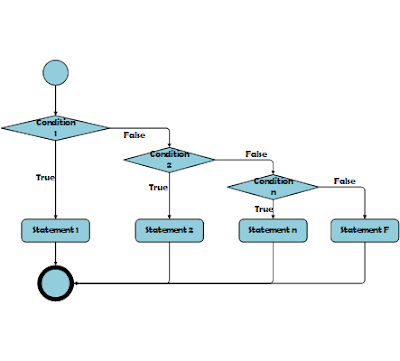### If-else Statement in C++

Decision making structures require that the programmer specify one or more conditions to be evaluated or tested by the program, along side a press release or statements to be executed if the condition is decided to be true, and optionally, other statements to be executed if the condition is decided to be false.

In C++ programming, if statement is employed to check the condition. There are various types of if statements in C++.

• if Statement
• if...else Statement
• nested if Statement
• if...else...if ladder

C++ If Statement

The C++ if statement tests the condition. It is executed if condition is true.

Syntax: -

if (condition){
//Code will be executed
}

Flow Chart: -If Statement

Example: -

#include <iostream>
using namespace std;

int main() {
if (10>5)
{
cout << "10 is Grater Than 5" ;
}
return 0;
}

When the above code is compiled and executed, it produces the following Output −

Output:
10 is Greater Than 5

C++ if...else Statement

An if statement are often followed by an optional else statement, which executes when the boolean expression is false.

Syntax: -

if (condition){
//true Code will be executed
}else{
//false Code will be executed
}

Flow Chart: -If...else Statement

Example: -

#include <iostream>
using namespace std;

int main() {
if (11 % 2 == 0)
{
cout << "It is Even";
}
else
{
cout << "It is Odd";
}
return 0;
}

When the above code is compiled and executed, it produces the following Output −

Output:
It is Odd

C++ Nested if...else Statement

Nested if else mean, you can create if statement inside another if statement.

Syntax: -

if (condition_1){
//Code will be executed if condition_1 true
if (condition_2){
//Code will be executed if condition_2 true
}
}

Flow Chart: -Nested if statement

Example: -

#include <iostream>
using namespace std;

int main() {
bool a = true;
bool b = true;
if (a)
{
if (b)
{
cout << "Both Condition are True";
}
}

return 0;
}

When the above code is compiled and executed, it produces the following Output −

Output:
Both Condition are True

C++ if...else Ladder Statement

The C++ if-else-if ladder statement executes many condition and execute multiple statements.

Syntax: -

if (condition_1){
//Code will be executed when condition_1 is true
}else if(condition_2){
//Code will be executed condition_2 is true
}else if(condition_3){
//Code will be executed condition_3 is true
}
...
else{
//Code will be executed all condition is false
}

Flow Chart: -If else ladder Statement

Example: -

#include <iostream>
using namespace std;

int main() {
int mark;
cout << "Enter Number:";
cin >> mark;
if (mark < 0 || mark > 100)
{
cout << "Wrong Number";
}
else if(mark >= 0 && mark <= 35)
{
cout << "Fail";
}
else if(mark >= 35 && mark <= 48)
{
cout << "D Grade";
}
else if(mark >= 50 && mark <= 64)
{
cout << "C Grade";
}
else if(mark >= 65 && mark <= 80)
{
cout << "B Grade";
}
else if(mark >= 81 && mark <= 100)
{
cout << "A Grade";
}
return 0;
}

When the above code is compiled and executed, it produces the following Output −

Output:
Enter Number
56
C Grade